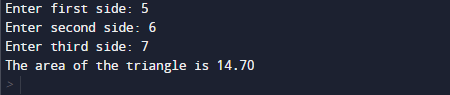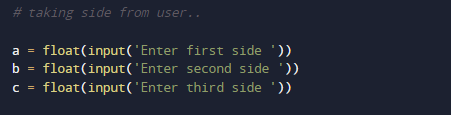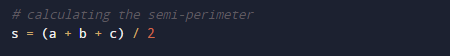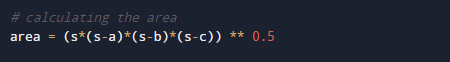# Python Program to Calculate the Area of a Triangle

In this tutorial you will learn about the Python Program to Calculate the Area of a Triangle and its application with practical example.

In this tutorial, we will learn to create a Python Program to Calculate the Area of a Triangle  using python Programming.

## Prerequisites

Before starting with this tutorial we assume that you are best aware of the following Python programming topics:

• Python Operator.
• Basic Input and Output
• Basic Python  programming.
• Python if-else statements.
• Python Loop.

## Area of a Triangle ?

Area of the triangle is going to be calculated by using ‘Heron’s formula’ gives the area of a triangle when the length of all three sides are known.## Python Program to Calculate the Area of a Triangle

In our program , we will calculate area of triangle.First we would declared and initialized the required variables. Next, we would prompt user to input height and width. Later we will find Area of a Triangle.

## Outputin our program, we have first declared and initialized a set variables required in the program.

• a= it will hold first value.
• b= it will hold second value.
• c= it will hold third value.
• s=for calculating the semi-perimeter
• area= it will hold area or traingle.

First of all  we declare variable and take three  values from user and assign them in ‘a’,’b’ and ‘c’ respectively.After taking these values from user we will calculate the semi-perimeter of Triangle.And after calculating the semi-perimeter we going to find the area of triangle using ‘Heron’s formula’  as follow.So we will use following steps to find area of Triangle.

• First ask the user to enter three lengths of a triangle.
• using Heron’s formula to calculate the semi-perimeter.
• Now  Area = (s*(s-a)*(s-b)*(s-c)) **0.5  to calculate the Area of the Triangle.At the end we will display the area we calculate area or Triangle.

In this tutorial we have learn about the Python Program to Calculate the Area of a Triangle and its application with practical example. I hope you will like this tutorial.